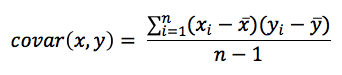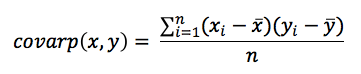# Covariance Functions

Covariance functions return the statistical covariance - how much two sets of values change together based on a sample of the population (COVAR), or based on a biased population (COVARP).

Covariance measures how two sets of values vary together. This elementary function is used as a building block for some of the two-dimensional statistical functions used in linear regression, as it describes collectively how the two sets of values vary around their mean values.

• COVAR (numeric1, numeric2)• where numeric1 and numeric2 are facts or metrics
• Computes the product of variance from mean for each value of x and y. These values are summed and then divided by (n].
• Use for sets of values that are only a sample of the total (biased) population.
• COVARP (numeric1, numeric2)• where numeric1 and numeric2 are facts or metrics
• Use covarp for calculating covariances when the numeric values are the entire population of values.
• Use for sets of values that include the entire (unbiased) population, use COVARP.

Syntax

``````SELECT COVAR(…)
SELECT COVAR(metric1, metric2)
SELECT COVAR(fact1, metric2)
SELECT COVAR(fact1, fact2)
SELECT COVARP(metric1, metric2)
SELECT COVARP(fact1, metric2)
SELECT COVARP(fact1, fact2)``````

Examples

``````SELECT COVAR(Total Cost of Inputs, Gross Margin)
SELECT COVARP(Height, Weight)``````